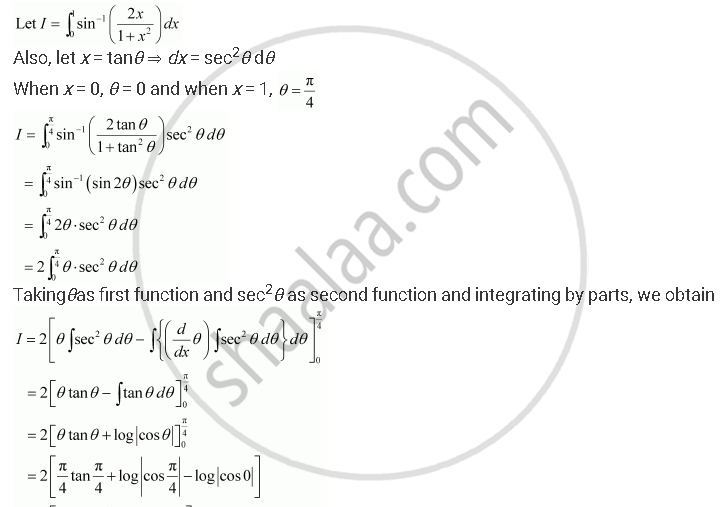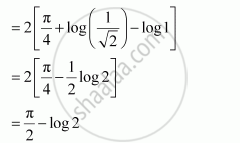Share

# Evaluate the Integrals in Using Substitution Int_0^1 Sin^(-1) ((2x)/(1+ X^2)) Dx - CBSE (Science) Class 12 - Mathematics

ConceptEvaluation of Definite Integrals by Substitution

#### Question

Evaluate the integrals in using substitution

int_0^1 sin^(-1) ((2x)/(1+ x^2)) dx

#### SolutionIs there an error in this question or solution?

#### Video TutorialsVIEW ALL 

Solution Evaluate the Integrals in Using Substitution Int_0^1 Sin^(-1) ((2x)/(1+ X^2)) Dx Concept: Evaluation of Definite Integrals by Substitution.
S